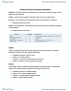# STAT151 Lecture Notes - Simple Random Sample, Standard Deviation, Sampling Distribution

52 views28 pages4
Verified Note
4 documents

## Document Summary

Definition: population parameter is a numerical measure such as the mean, median, mode, range, variance, or standard deviation calculated for a population data; and is written with greek letters. Usually unknown and constant: sample statistic is a summary measure calculated for a sample data set; it is written with latin letters. Regarded as random before sample is selected. Observed after sample is selected: the value of the statistic varies from sample to sample, this is called sampling variability, the distribution of all the values of a statistic is called its sampling distribution. Suppose we are just interested in one characteristic occurred in the population of interest. For convenience, we will call the outcome we are looking for success (s). 1 of 28 sy,is obtained by taking the ratio of the number of successes in a population to the total number of elements in the population. Check for n students, how many are nonresidents.

## Get access

\$8 USD/m\$10 USD/m
Billed \$96 USD annuallyHomework Help
Study Guides
Textbook Solutions
Class Notes
Textbook Notes
Booster Class
Class+
\$8 USD/m
Billed \$96 USD annuallyHomework Help
Study Guides
Textbook Solutions
Class Notes
Textbook Notes
Booster Class